Pull Down & Release to Refresh

# Gradient of a Straight Line

The gradient of a straight line is a number that tells you how steep its slope is. Another word for gradient is slope.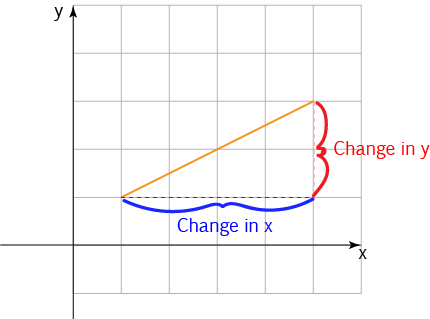The gradient is given by the following formula:

$\text{Gradient} = \dfrac{\text{Change in } y}{\text{Change in } x}$
So, we divide the change in the height of the line by the change in the horizontal distance it covers.

## Vertical Rise Over Horizontal Run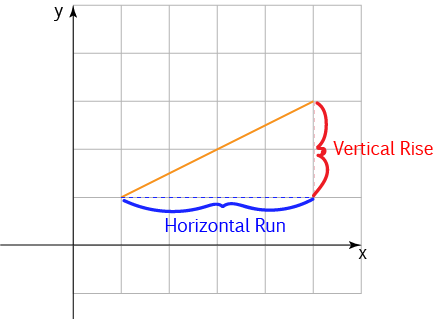We often call the change in height the vertical rise, and the change in horizontal distance the horizontal run, so the formula for the gradient can be written as :

$\text{Gradient} = \dfrac{\text{Vertical Rise}}{\text{Horizontal Run}}$
This gives exactly the same answer, it's just a change of terminology for the same things.

### Example 1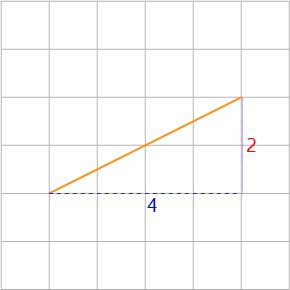The vertical rise is $2$ and the horizontal run is $4$, so the gradient is

$\text{Gradient} = \dfrac{2}{4} = \dfrac{1}{2}$

### Example 2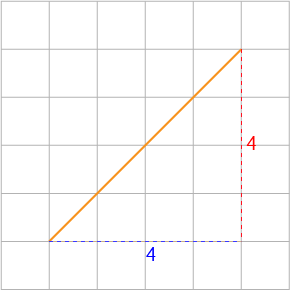The vertical rise is $4$ and the horizontal run is $4$, so the gradient is

$\text{Gradient} = \dfrac{4}{4} = 1$

Did you notice that this line is steeper than the line in Example 1? Its gradient is larger, too.

### Example 3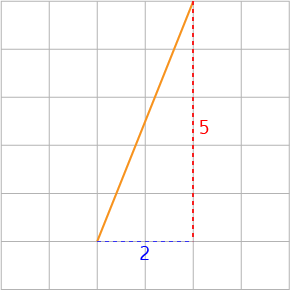The vertical rise is $5$ and the horizontal run is $2$, so the gradient is

$\text{Gradient} = \dfrac{5}{2} = 2.5$

This line is even steeper than the line in Example 2. Its gradient is larger, too.

Gradients can be positive, negative or zero.

• When the gradient is positive, the straight line is going uphill as we move from left to right. We say that the straight line function is increasing.
• When the gradient is negative, the straight line is going downhill as we move from left to right. We say that the straight line function is decreasing.
• When the gradient is zero, the straight line is horizontal. It slopes neither uphill nor downhill.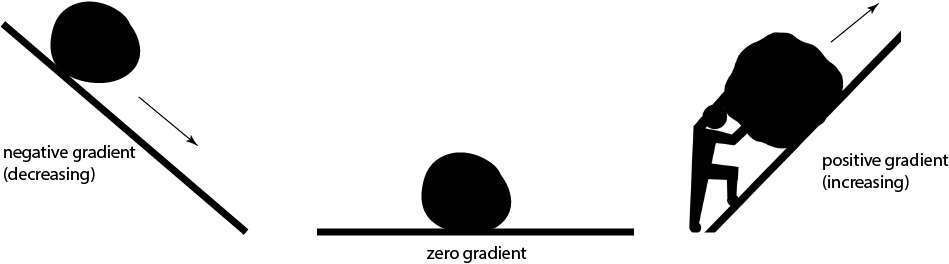In the picture, when the gradient is negative, the boulder can roll down the hill. When the gradient is zero, the boulder just sits on the flat. When the gradient is positive, the man has to push the boulder up the hill.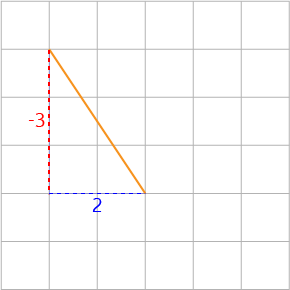The vertical rise is $-3$ as the line is sloping downwards, and the horizontal run is $2$, so the gradient is

$\text{Gradient} = \dfrac{-3}{2} = -1.5$

This line is sloping downhill, so it has a negative gradient.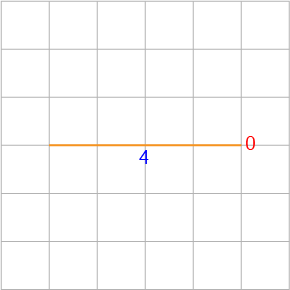The vertical rise is $0$ as the line is horizontal (flat), and the horizontal run is $4$, so the gradient is

$\text{Gradient} = \dfrac{0}{4} = 0$

This line is horizontal, so its gradient is zero.

### Example: Vertical LineThe vertical rise is $4$, and the horizontal run is $0$ as the line is vertical, so the gradient is

$\text{Gradient} = \dfrac{4}{0} = ?$

That's a bit of a problem, isn't it? We say that the gradient of vertical lines is undefined.

## Summary

The gradient of a line that slopes uphill is positive.

The gradient of a line that slopes downhill is negative.

The gradient of a horizontal line is zero.

The gradient of a vertical line is undefined.

Steeper (uphill) lines have larger gradients.

Steeper downhill lines have negative gradients that have larger sizes.

### Description

coordinate geometry is a branch of geometry where the position of the points on the plane is defined with the help of an ordered pair of numbers also known as coordinates. In this tutorial series, you will learn about vast range of topics such as Cartesian Coordinates, Midpoint of a Line Segment etc

### Audience

year 10 or higher, several chapters suitable for Year 8+ students.

### Learning Objectives

Explore topics related to Coordinates Geometry

Author: Subject Coach
You must be logged in as Student to ask a Question.